1642 Isaac Newton was born on 25 December.Light | Alchemy | Motion | Notebooks

He was most influenced by the 1634 book,

The Mysteries of Nature and Art

1666

Infinitesimals

Calculus

lucasian Professor

Prisms

Reveal that white light is actually a composite of colors.

Colors

·       r

·       o

·       y

·       g

·       b

·       i

·       v

From lowest to highest frequency we see: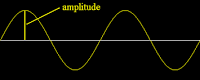Red

Orange

Yellow

Green

Blue

Indigo

Violet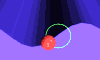Most of what Newton's notebooks contain with respect to science had to deal with his alchemical experiments.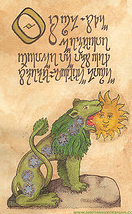Alchemy - early modem chemistry

Philosopher's stone

Alchemical recipes

Ø  Hermaphrodite is the mother & father of the stone

Ø  Green lion

Ø  Sordid whore

Ø  Copper, iron & fire

The purple alloy is the net in which Venus and Mars are captured

Keynes bought Newton's papers in the 1930s and in them we discovered many previously overlooked ideas.

Newton was convinced that the Trinity was not true; he denied the divinity of Christ as a violation of the first commandment.

God is imminent in the universe

Created by the "counsel and dominion of God"

He calculated that in 980 BC civilization began and Christ will return in 2060.

Sir Edmund Halley went to see Newton and Newton told him he had worked out the formula for the Ellipse

inverse square law

means there are elliptical orbits of planets about the sun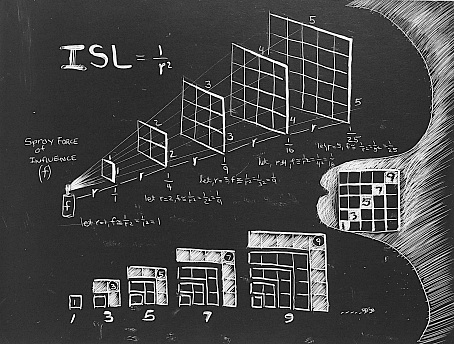Three laws of motion  for every action there is an equal and opposite reaction

An object in motion will remain in motion or at rest.

1687 Principia Mathematica

The parabolic path of motion

Newton's First Law of Motion:

 I. Every object in a state of uniform motion tends to remain in that state of motion unless an external force is applied to it.

This we recognize as essentially Galileo's concept of inertia, and this is often termed simply the "Law of Inertia".

Newton's Second Law of Motion:

 II. The relationship between an object's mass m, its acceleration a, and the applied force F is F = ma. Acceleration and force are vectors (as indicated by their symbols being displayed in slant bold font); in this law the direction of the force vector is the same as the direction of the acceleration vector.

This is the most powerful of Newton's three Laws, because it allows quantitative calculations of dynamics: how do velocities change when forces are applied. Notice the fundamental difference between Newton's 2nd Law and the dynamics of Aristotle: according to Newton, a force causes only a change in velocity (an acceleration); it does not maintain the velocity as Aristotle held.

This is sometimes summarized by saying that under Newton, F = ma, but under Aristotle F = mv, where v is the velocity. Thus, according to Aristotle there is only a velocity if there is a force, but according to Newton an object with a certain velocity maintains that velocity unless a force acts on it to cause an acceleration (that is, a change in the velocity). As we have noted earlier in conjunction with the discussion of Galileo, Aristotle's view seems to be more in accord with common sense, but that is because of a failure to appreciate the role-played by frictional forces. Once account is taken of all forces acting in a given situation it is the dynamics of Galileo and Newton, not of Aristotle, that are found to be in accord with the observations.

Newton's Third Law of Motion:

 III. For every action there is an equal and opposite reaction.

Theology

Newton was a Unitarian as he rejected the notion of the trinity.

2060 is the date Newton determined will be the second coming of Jesus Christ.

Opticks,  1702

He died in 1727 at 84 years old.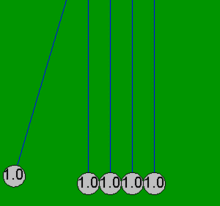Every object in a state of uniform motion tends to remain in a state of motion unless acted upon by an applied external force.

Light | Alchemy | Motion | Notebooks

Sir Edmond Halley